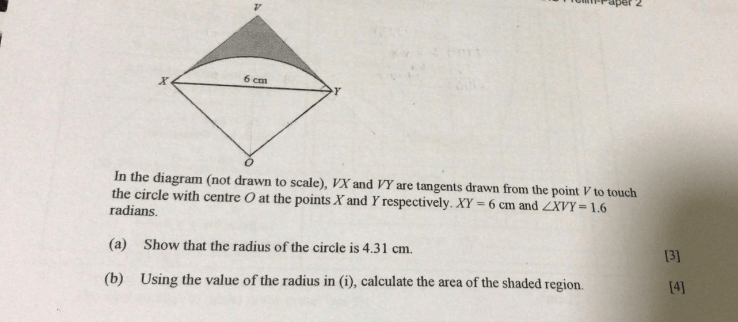# QuestionHi, can anyone help me with the question?

Thanks in advance!

1 Answer

# Answer

(a)

VX = VY (tangent properties)

So, OXVY is a kite (OX=OY, VX=VY)

Draw a line to join OV.

OV is the perpendicular bisector of XY (geometric properties of kite)

Name the midpoint of XY , M

Then MX=MY = 3cm and

∠MVY =  ∠MVX = 0.8 rad

∠MVY + ∠MYV

= ∠MYO + ∠MYV = 90°

∴ ∠MYO = ∠MVY = 0.8 rad

Radius, OY = MY/cos(∠MYO)

= 3 cm/ cos(0.8 rad)

= 4.3059… = 4.31 (3 s.f.)

I trust that you can work out (b)

cheers.

0 Replies 0 Likes